## Convergence, Divergence, and Comparison

In this section we discuss using comparison to determine if an improper integrals converges or diverges. Recall that if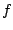and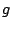are continuous functions on an interval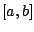and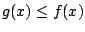, then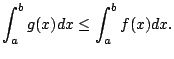This observation can be incredibly useful in determining whether or not an improper integral converges.

Not only does this technique help in determing whether integrals converge, but it also gives you some information about their values, which is often much easier to obtain than computing the exact integral.

Theorem 5.7.8 (Comparison Theorem (special case))   Letandbe continuous functions with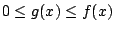for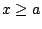.
1. If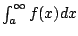converges, thenconverges.
2. Ifdiverges thendiverges.

Proof. Since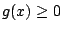for all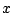, the function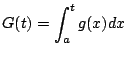is a non-decreasing function. Ifconverges to some value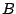, then for any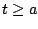we have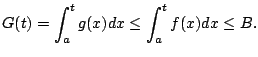Thus in this case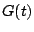is a non-decreasing function bounded above, hence the limit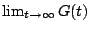exists. This proves the first statement.

Likewise, the function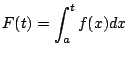is also a non-decreasing function. Ifdiverges then the functiondefined above is still non-decreasing anddoes not exist, sois not bounded. Sincewe have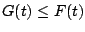for all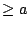, hence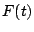is also unbounded, which proves the second statement.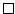The theorem is very intuitive if you think about areas under a graph. If the bigger integral converges then so does the smaller one, and if the smaller one diverges so does the bigger ones.''

Example 5.7.9   Does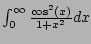converge? Answer: YES.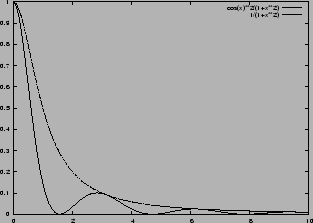Since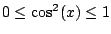, we really do have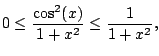as illustrated in Figure 5.7.5. Thus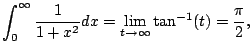soconverges.

But why did we use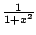? It's a guess that turned out to work. You could have used something else, e.g.,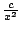for some constant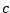. This is an illustration of how in mathematics sometimes you have to use your imagination or guess and see what happens. Don't get anxious--instead, relax, take a deep breath and explore.

For example, alternatively we could have done the following: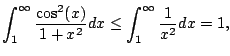and this works just as well, since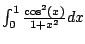converges (as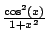is continuous).

Example 5.7.10   Consider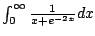. Does it converge or diverge? For large values of, the term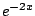very quickly goes to 0, so we expect this to diverge, since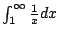diverges. For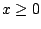, we have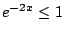, so for allwe have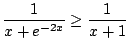(verify by cross multiplying)But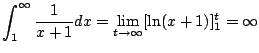Thusmust also diverge.

Note that there is a natural analogue of Theorem 5.7.8 for integrals of functions that blow up'' at a point, but we will not state it formally.

Example 5.7.11   Consider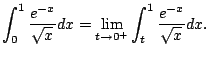We have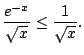(Coming up with this comparison might take some work, imagination, and trial and error.) We have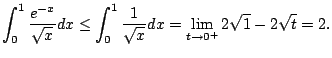thus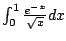converges, even though we haven't figured out its value. We just know that it is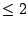. (In fact, it is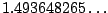.)

What if we found a function that is bigger than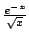and its integral diverges?? So what! This does nothing for you. Bzzzt. Try again.

Example 5.7.12   Consider the integral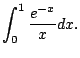This is an improper integral since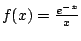has a pole at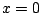. Does it converge? NO.
On the interal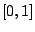we have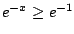. Thus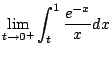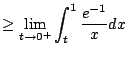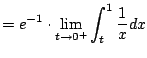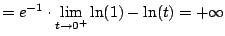Thus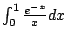diverges.

William Stein 2006-03-15